实现有序排列的多空均衡权益策略

Author: , Created: 2019-08-24 10:05:53, Updated: 2019-09-01 00:27:48

怎样制定排名方案？

• 你将投资标的排列为:1,…,p这样的位置，做空2/2p美元的投资标的

• 你将投资标的排列为:m-p,…,m这样的位置，做多n/2p美元的投资标的

**注意：**由于价格跳动导致的投资标的价格不会总是均匀地划分n/2p，并且必须以整数购买某些投资标的，所以会有一些不精确的算法，算法应尽可能接近这个数字。对于运行n = 100000和p = 500的策略，我们看到:

n/2p = 100000/1000 = 100

让我们来看一个假设的例子吧

• 在发明者量化平台搭建我们的研究环境

import numpy as np
import statsmodels.api as sm
import scipy.stats as stats
import scipy
import matplotlib.pyplot as plt
import seaborn as sns
import pandas as pd
## PROBLEM SETUP ##
# Generate stocks and a random factor value for them
stock_names = ['stock ' + str(x) for x in range(10000)]
current_factor_values = np.random.normal(0, 1, 10000)
# Generate future returns for these are dependent on our factor values
future_returns = current_factor_values + np.random.normal(0, 1, 10000)
# Put both the factor values and returns into one dataframe
data = pd.DataFrame(index = stock_names, columns=['Factor Value','Returns'])
data['Factor Value'] = current_factor_values
data['Returns'] = future_returns
# Take a look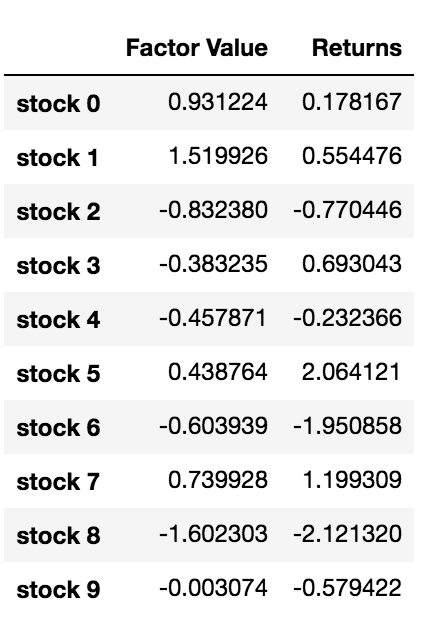# Rank stocks
ranked_data = data.sort_values('Factor Value')
start = i * 500
end = i * 500 + 500
# Plot the returns of each basket
plt.figure(figsize=(15,7))
plt.ylabel('Returns')
plt.show()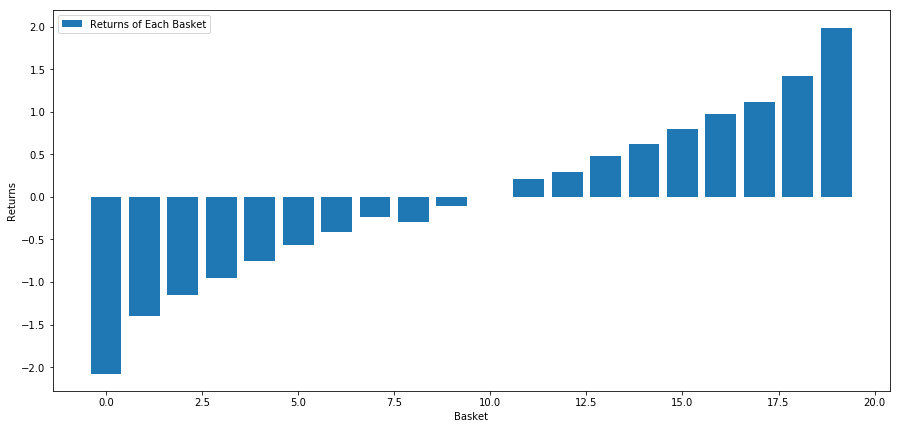让我们考虑一个现实世界的例子。

from backtester.dataSource.yahoo_data_source import YahooStockDataSource
from datetime import datetime
startDateStr = '2010/01/01'
endDateStr = '2017/12/31'
cachedFolderName = '/Users/chandinijain/Auquan/yahooData/'
instrumentIds = ['ABT','AKS','AMGN','AMD','AXP','BK','BSX',
'CMCSA','CVS','DIS','EA','EOG','GLW','HAL',
'HD','LOW','KO','LLY','MCD','MET','NEM',
'PEP','PG','M','SWN','T','TGT',
'TWX','TXN','USB','VZ','WFC']
ds = YahooStockDataSource(cachedFolderName=cachedFolderName,
dataSetId=dataSetId,
instrumentIds=instrumentIds,
startDateStr=startDateStr,
endDateStr=endDateStr,
event='history')

## Define normalized momentum
## Load relevant prices in a dataframe
#Let's load momentum score and returns into separate dataframes
index = data.index
mscores = pd.DataFrame(index=index,columns=assetList)
mscores = momentum(data, 30)
returns = pd.DataFrame(index=index,columns=assetList)
day = 30

分析数据

# Calculate Forward returns
forward_return_day = 5
returns = data.shift(-forward_return_day)/data -1
returns.dropna(inplace = True)
# Calculate correlations between momentum and returns
correlations = pd.DataFrame(index = returns.columns, columns = [‘Scores’, ‘pvalues’])
mscores = mscores[mscores.index.isin(returns.index)]
for i in correlations.index:
score, pvalue = stats.spearmanr(mscores[i], returns[i])
correlations[‘pvalues’].loc[i] = pvalue
correlations[‘Scores’].loc[i] = score
correlations.dropna(inplace = True)
correlations.sort_values(‘Scores’, inplace=True)
l = correlations.index.size
plt.figure(figsize=(15,7))
plt.bar(range(1,1+l),correlations[‘Scores’])
plt.xlabel(‘Stocks’)
plt.xlim((1, l+1))
plt.xticks(range(1,1+l), correlations.index)
plt.legend([‘Correlation over All Data’])
plt.ylabel(‘Correlation between %s day Momentum Scores and %s-day forward returns by Stock’%(day,forward_return_day));
plt.show()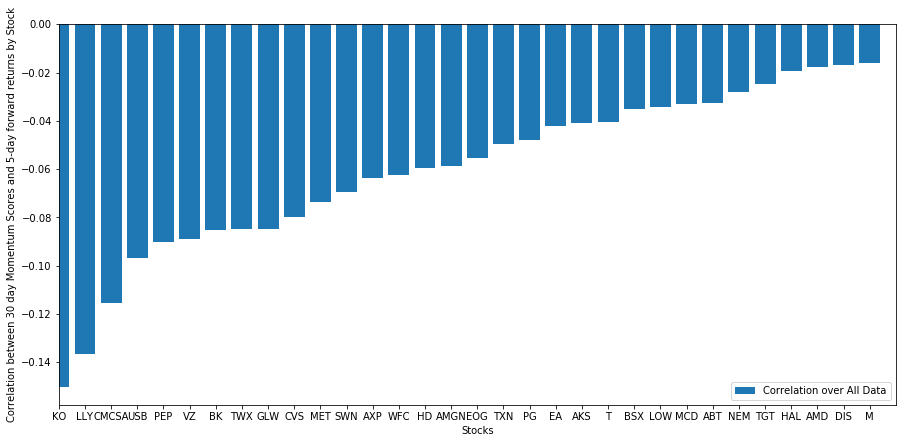动量分析得分排序与收益之间的相关性

correl_scores = pd.DataFrame(index = returns.index.intersection(mscores.index), columns = [‘Scores’, ‘pvalues’])
for i in correl_scores.index:
score, pvalue = stats.spearmanr(mscores.loc[i], returns.loc[i])
correl_scores[‘pvalues’].loc[i] = pvalue
correl_scores[‘Scores’].loc[i] = score
correl_scores.dropna(inplace = True)
l = correl_scores.index.size
plt.figure(figsize=(15,7))
plt.bar(range(1,1+l),correl_scores[‘Scores’])
plt.hlines(np.mean(correl_scores[‘Scores’]), 1,l+1, colors=’r’, linestyles=’dashed’)
plt.xlabel(‘Day’)
plt.xlim((1, l+1))
plt.legend([‘Mean Correlation over All Data’, ‘Daily Rank Correlation’])
plt.ylabel(‘Rank correlation between %s day Momentum Scores and %s-day forward returns’%(day,forward_return_day));
plt.show()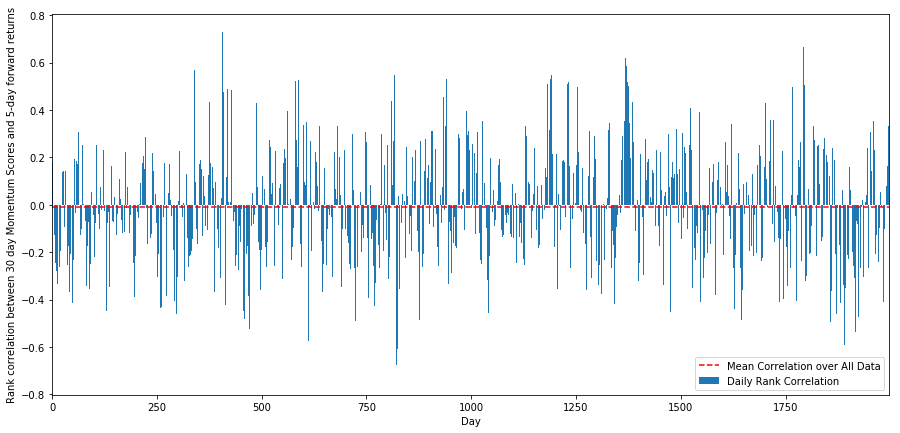monthly_mean_correl =correl_scores['Scores'].astype(float).resample('M').mean()
plt.figure(figsize=(15,7))
plt.bar(range(1,len(monthly_mean_correl)+1), monthly_mean_correl)
plt.hlines(np.mean(monthly_mean_correl), 1,len(monthly_mean_correl)+1, colors='r', linestyles='dashed')
plt.xlabel('Month')
plt.xlim((1, len(monthly_mean_correl)+1))
plt.legend(['Mean Correlation over All Data', 'Monthly Rank Correlation'])
plt.ylabel('Rank correlation between %s day Momentum Scores and %s-day forward returns'%(day,forward_return_day));
plt.show()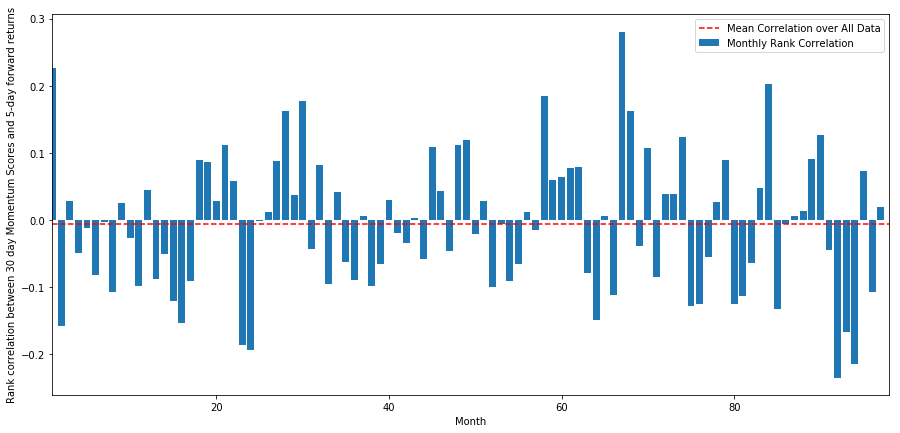平均一篮子股票回报率

data = pd.concat([factor.loc[index],forward_returns.loc[index]], axis=1)
# Rank the equities on the factor values
data.columns = ['Factor Value', 'Forward Returns']
data.sort_values('Factor Value', inplace=True)
# How many equities per basket
# Compute the returns of each basket
if i == number_of_baskets - 1:
# Handle having a few extra in the last basket when our number of equities doesn't divide well
end = len(data.index) - 1
else:
# Actually compute the mean returns for each basket
#s = data.index.iloc[start]
#e = data.index.iloc[end]

resampled_scores = mscores.astype(float).resample('2D').last()
resampled_prices = data.astype(float).resample('2D').last()
resampled_scores.dropna(inplace=True)
resampled_prices.dropna(inplace=True)
forward_returns = resampled_prices.shift(-1)/resampled_prices -1
forward_returns.dropna(inplace = True)
for m in forward_returns.index.intersection(resampled_scores.index):
# Plot the returns of each basket
plt.figure(figsize=(15,7))
plt.ylabel('Returns')
plt.show()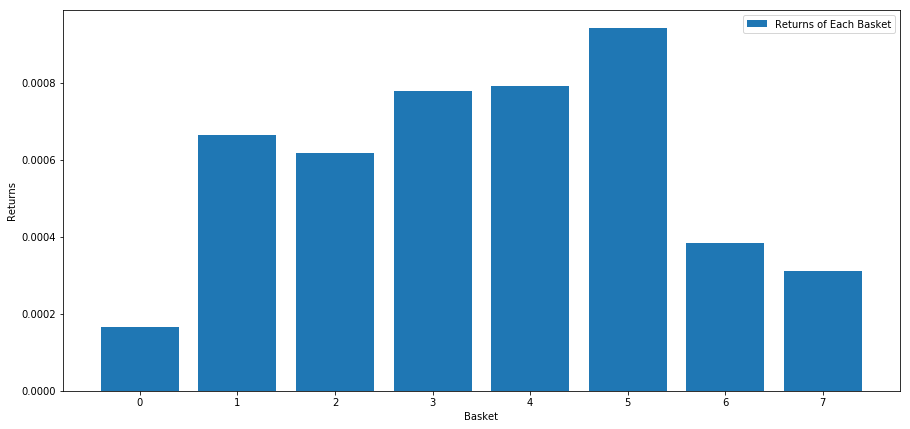利差(基差)一致性

total_months = mscores.resample('M').last().index
months_to_plot = 24
monthly_index = total_months[:months_to_plot+1]
strategy_returns = pd.Series(index = monthly_index)
f, axarr = plt.subplots(1+int(monthly_index.size/6), 6,figsize=(18, 15))
for month in range(1, monthly_index.size):
temp_returns = forward_returns.loc[monthly_index[month-1]:monthly_index[month]]
temp_scores = resampled_scores.loc[monthly_index[month-1]:monthly_index[month]]
for m in temp_returns.index.intersection(temp_scores.index):

r = int(np.floor((month-1) / 6))
c = (month-1) % 6
axarr[r, c].xaxis.set_visible(False)
axarr[r, c].set_title('Month ' + str(month))
plt.show()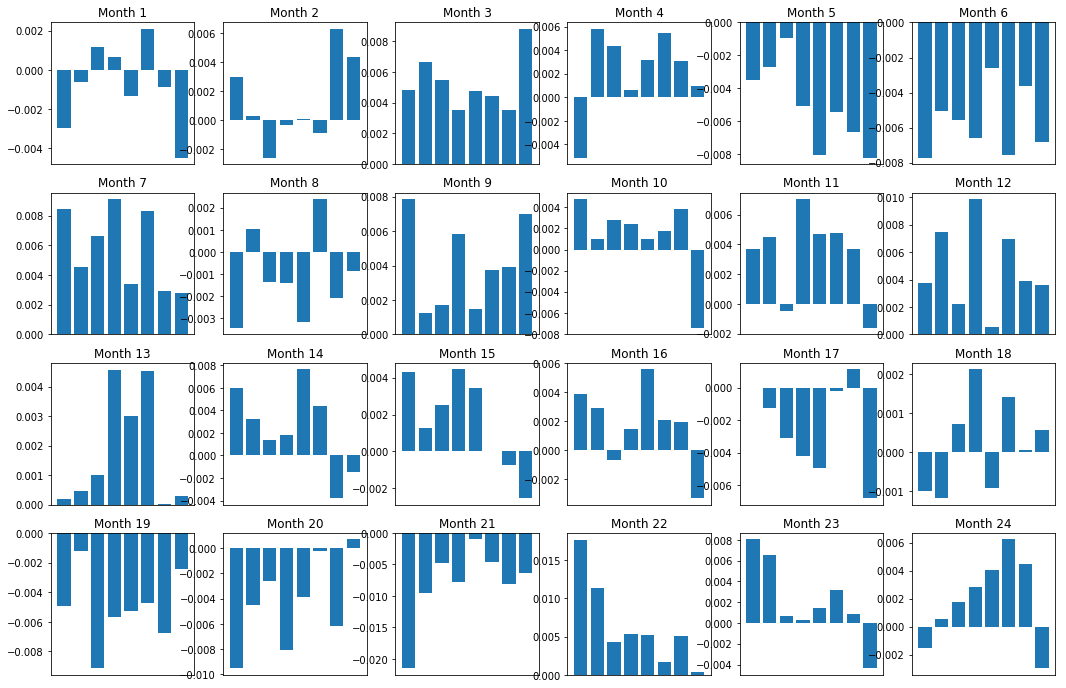plt.figure(figsize=(15,7))
plt.plot(strategy_returns)
plt.ylabel(‘Returns’)
plt.xlabel(‘Month’)
plt.plot(strategy_returns.cumsum())
plt.legend([‘Monthly Strategy Returns’,’Cumulative Strategy Returns’])
plt.show()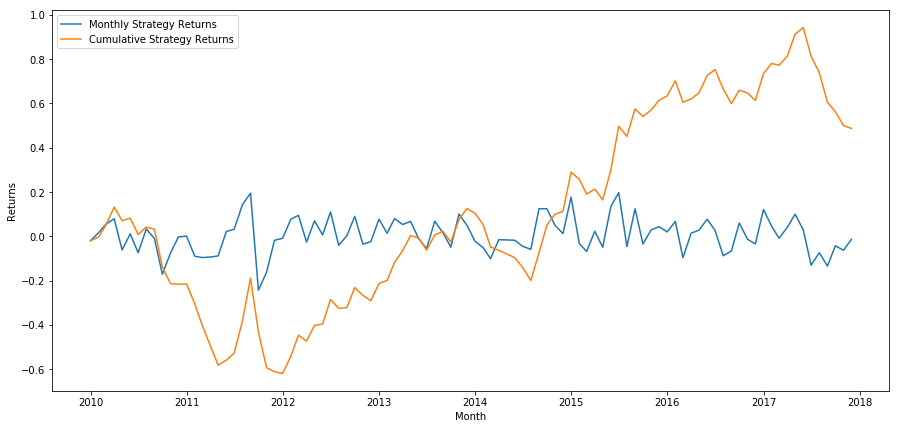total_return = strategy_returns.sum()
ann_return = 100*((1 + total_return)**(12.0 /float(strategy_returns.index.size))-1)
print('Annual Returns: %.2f%%'%ann_return)

找到正确的排名方案

排名方案的选择与评估

• 克隆和调整：选择一个经常讨论的内容，看看是否可以稍微修改它以获得优势。通常情况下，公开的因子将不再有交易信号，因为它们已完全套利出市场。但是，有时它们会引导你朝着正确的方向前进。

• 定价模型：任何预测未来回报的模型都可能是一个因子,都有潜在的可能用于对你的一篮子交易标的进行排名。你可以采用任何复杂的定价模型并将其转换为排名方案。

• 基于价格的因子（技术指标）：基于价格的因子，如我们今天所讨论的，获取有关每种权益的历史价格的信息，并使用它来生成因子价值。例子可能是移动平均指标，动量指标或波动率指标。

• 回归与动量：值得注意的是，有些因子认为价格一旦朝着一个方向发展，就会继续这样做。有些因子恰恰相反。两者都是关于不同时间范围和资产的有效模型，并且研究基础行为是基于动量还是基于回归是很重要的。

• 基本因子（基于价值）：这是使用基本价值的组合，如PE，股息等。基本价值包含与公司的现实世界事实相关的信息，因此在许多方面可以比价格更强大。

• 重新平衡频率

• 资本能力和交易成本

More